Courses

# Match the Following of Modern Physics, Past year Questions JEE Advance, Class 12, Physics JEE Notes | EduRev

## JEE : Match the Following of Modern Physics, Past year Questions JEE Advance, Class 12, Physics JEE Notes | EduRev

The document Match the Following of Modern Physics, Past year Questions JEE Advance, Class 12, Physics JEE Notes | EduRev is a part of the JEE Course Class 12 Physics 35 Years JEE Mains &Advance Past year Paper.
All you need of JEE at this link: JEE

DIRECTIONS (Q. No. 1 to 4 & 6) : Each question contains statements given in two columns, which have to be matched. The statements in Column-I are labelled A, B, C and D, while the statements in Column-II are labelled p, q, r and s. Any given statement in Column-I can have correct matching with ONE OR MORE statement(s) in Column-II. The appropriate bubbles corresponding to the answers to these questions have to be darkened as illustrated in the following example :

If the correct matches are A-p, s and t; B-q and r; C-p and q; and D-s then the correct darkening of bubbles will look like the given.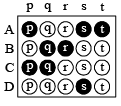Q.1. In the following, column I lists some physical quantities and the column II gives approximate energy values associated with some of them. Choose the appropriate value of energy from column II for each of the physical quantities in column I and write the corresponding letter p, q, r, etc. against the number (A), (B), (C), (D) etc. of the physical quantity in the answer book. In your answer, the sequence of column I should be maintained.          (1997 - 4 Marks)

 Column I Column II (A) Energy of thermal neutrons (p) 0.025 eV (B) Energy of X-rays (q) 0.5 eV (C) Binding energy per nucleon (r) 3 eV (D) Photoelectric threshold of a metal (s) 20 eV (t) 10 k eV (u) 8 M eV

Ans. A-p; B-t; C-u; D-r

Solution. A → p; B → t; C → u; D → r

The correct match is as follows :

(A) Energy of thermal neutrons                  (p) 0.025 eV
(B) Energy of X-rays                                     (t) 10 k eV
(C) Binding energy per nucleon                  (u) 8 M eV
(D) Photoelectric threshold                         (r) 3 eV of a metal

Q.2. Given below are certain matching type questions, where two columns (each having 4 items) are given. Immediately after the columns the matching grid is given, where each item of Column I has to be matched with the items of Column II, by encircling the correct match(es). Note that an item of column I can match with more than one item of column II. All the items of column II must be matched. Match the following :     (2006 - 6M)

 Column I Column II (A) Nuclear fusion (p) Converts some matter into energy (B) Nuclear fission (q) Generally possible for nuclei with low atomic number (C) β-decay (r) Generally possible for nuclei with higher atomic number (D) Exothermic nuclear reaction (s) Essentially proceeds by weak nuclear forces

Ans. A-p, q; B-p, r; C-p, s; D-p, q, r

Solution.  A → p, q; B → p, r; C → p, s; D → p, q, r

In a nuclear fusion reaction matter is converted into energy and nuclei of low atomic number generally given this reaction.

In a nuclear fission reaction matter is converted into energy and nuclei of high atomic number generally given this reaction.

Q.3. Some laws / processes are given in Column I. Match these with the physical phenomena given in Column II and indicate your answer by darkening appropriate bubbles in the 4 × 4 matrix given in the ORS.

 Column I Column II (A) Transition between two atomic energy levels (p) Characteristic X-rays (B) Electron emission from a material (q) Photoelectric effect (C) Mosley's law (r) Hydrogen spectrum (D) Change of photon energy into kinetic energy of electrons (s) β-decay

Ans. A-p, r; B-q, s; C-p; D-q

Solution. A → p, r

Reason : Characteristic X-ray are produced due to transition of electrons from one energy level to another.

Similarly the lines in the hydrogen spectrum is obtained due to transition of electrons from one energy level to another.

B → q, s

Reason : In photoelectric effect electrons from the metal surface are emitted out upon the incidence of light of appropriate frequency.

Note : In β-decay, electrons are emitted from the nucleus of an atom.

C → p

Moseley gave a law which related frequency of emitted Xray with the atomic number of the target material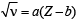D → q

In photoelectric effect, energy of photons of incident ray gets converted into kinetic energy of emitted electrons.

Q.4. Column-II gives certain systems undergoing a process. Column-I suggests changes in some of the parameters related to the system. Match the statements in Column-I to the approapriate process(es) from Column-II.

 Column-I Column-II (A) The energy of the system is increased (p) System : A capacitor, initially uncharged Process : It is connected to a battery (B) Mechanical energy is provided to the system, which is converted into energy of random motion of its parts (q) System : A gas in an adiabatic container fitted with an adiabatic pistonProcess: The gas is compressed by pushing the piston (C) Internal energy of the system is converted into its mechanical energy (r) System: A gas in a rigid containerProcess: The gas gets cooled due to colder atmosphere surrounding it (D) Mass of the system is decreased (s) System: A heavy nucleus, initially at restProcess: The nucleus fissions into two fragments of nearly equal masses and some neutrons are emitted (t) System: A resistive wire loopProcess: The loop is placed in a time varying magnetic field perpendicular to its plane

Ans. A-p, q, t; B-q; C-s; D-s

Solution. A → p, q, t; B → q; C → s; D → s

(p) When an uncharged capacitor is connected to a battery, it becomes charged and energy is stored in the capacitor. (A) is the correct option.

(q) When a gas in an adiabatic container fitted with an adiabatic piston is compressed by pushing the piston

(i) the internal energy of the system increases

ΔU = Q - W = 0 - (- PdV) =+ PdV

(ii) Mechanical energy is proceeded to the piston which is converted into kinetic energy of the gas molecules.
(r) None of the options in column I matches. As the gas in a rigid container gets cooled, the internal energy of the system will decrease. The average kinetic energy per molecule will decrease.
(s) When a heavy nucleus initially at rest splits into two nuclei of nearly equal masses  and some neutrons are emitted then
(i) Internal energy of the system is converted into mechanical energy (precisely speaking kinetic energy) and
(ii) Mass of the system decr eases which converts into energy.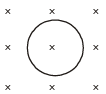(t) When a resistive wire loops is placed in a time varying magnetic field perpendicular to its palne.
(i) Induced current shows in the loop due to which the energy of system is increased.

DIRECTION (Q.No. 5) : Following question has matching list I and II. The codes for the lists have choices (a), (b), (c) and (d) out of which ONLY ONE is correct.

Q.5. Match List I of the nuclear processes with List II containing parent nucleus and one of the end products of each process and then select the correct answer using the codes given below the lists:                 (JEE Adv. 2013-II)

 List I List II P. Alpha decay 1.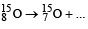Q. b+ decay 2.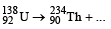R. Fission 3.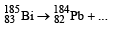S. Proton emission 4.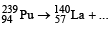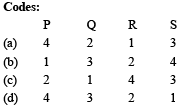Ans. (c)

Solution.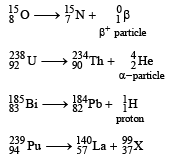(c) is the correct option.

Q.6. Match the nuclear processes given in column I with the appropriate option(s) in column II.                      (JEE Adv. 2015)

 Column I Column II (A) Nuclear fusion (p) Absorption of thermal neutrons by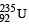(B) Fission in a nuclear reactor (q)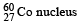(C) β-decay (r) Energy production in stars via hydrogen conversion to helium (D) γ-ray emission (s) Heavy water (t) Neutrino emission

Ans. A-r, t; B-p, s; C-p, q, r, t; D-p, q, r, t

Solution. A → r, t; B → p, s; C → p, q, r, t; D → p, q, r, t

Based on facts

Offer running on EduRev: Apply code STAYHOME200 to get INR 200 off on our premium plan EduRev Infinity!

42 docs|19 tests

,

,

,

,

,

,

,

,

,

,

,

,

,

,

,

,

,

,

,

,

,

,

,

,

,

,

,

,

,

,

;